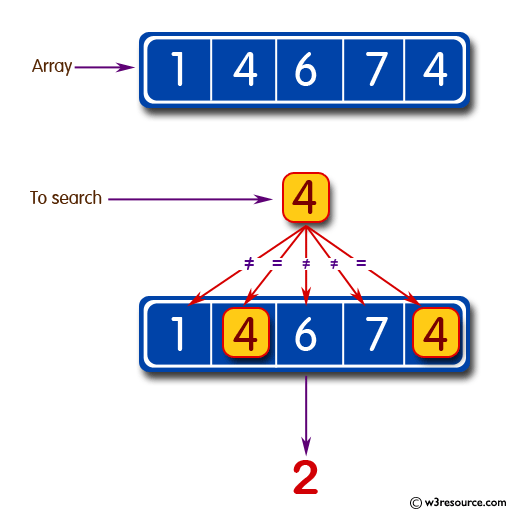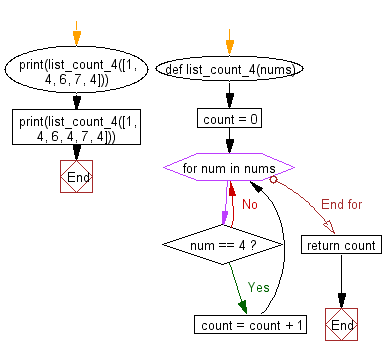﻿ Python: Count the number 4 in a given list - w3resource# Python: Count the number 4 in a given list

## Python Basic: Exercise-22 with Solution

Write a Python program to count the number 4 in a given list.

Pictorial Presentation:Sample Solution:-

Python Code:

``````def list_count_4(nums):
count = 0
for num in nums:
if num == 4:
count = count + 1

return count

print(list_count_4([1, 4, 6, 7, 4]))
print(list_count_4([1, 4, 6, 4, 7, 4]))```
```

Sample Output:

```2
3
```

Flowchart:## Visualize Python code execution:

The following tool visualize what the computer is doing step-by-step as it executes the said program:

Python Code Editor:

Have another way to solve this solution? Contribute your code (and comments) through Disqus.

What is the difficulty level of this exercise?

Test your Python skills with w3resource's quiz

﻿

## Python: Tips of the Day

Unpack variables from iterable:

```# One can unpack all iterables (tuples, list etc)
>>> a, b, c = 1, 2, 3
>>> a, b, c
(1, 2, 3)

>>> a, b, c = [1, 2, 3]
>>> a, b, c
(1, 2, 3)
```